If you are thinking that nobody in their right mind would implement a Calculator API Service with a machine learning model, then yes, you’re probably right. But considering curiosity is in my DNA, it sometimes works this way and machine learning is fun. I have challenged myself to do it, not for the purpose of promoting such an experiment into production, but simply accomplishing a self-challenge that can be easily achieved with the resources provided by SAS Viya, particularly SAS Model Studio and SAS Intelligent Decisioning.

So, first, let’s define the purpose of my challenge:

### deploy a basic calculator API service capable of executing the following operations for two input decimal numbers: addition, subtraction, multiplication, and division

The challenge must be executed under these restrictions:

• Usage of a machine learning model as the “compute-engine” of the calculator
• Development under the low code / no code paradigm
• The end-to-end setup and execution process should not take more than a couple of hours

Use the following tools in SAS Viya:

• Model Studio
• Intelligent Decisioning
• Simple web app (set up not covered)

# The plan

The steps that I am identifying to complete my challenge are:
Step 1 - Create a machine learning model representing the compute-engine of my calculator (Model Studio)
Step 2 - Determine how to process other mathematical operations
Step 3 - Step 3 - Embed the needed logic into the decision to perform the calculator operations (Intelligent Decisioning)
Step 4 - Publish the artifact created as an API service (web app created outside of this post)

## Step 1. Create a machine learning model as the compute-engine

Our first step is to create a model. We start with the addition operation and build from there. We’ll perform the addition by normal means of adding two numbers. Next, we’ll apply some extra logic which will perform subtraction, multiplication, and division. The diagram below represents the process: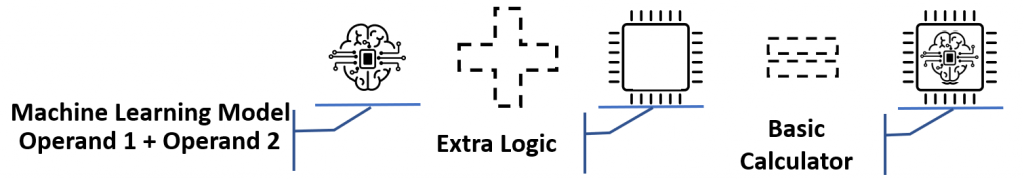A machine learning model is built from a data set where it self-learns what to do. I want my model to learn the addition of two numbers. I created a training data set in Excel with 100 registers, each of them with two random numbers between 0 and 1 and then the sum of them. The image below displays the general setup: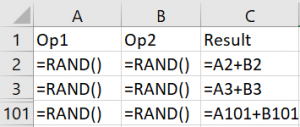The algorithm / model I chose for my compute engine is the linear regression. Linear regression is a simple machine learning algorithm based in the following formula:

y = a + X1 + c·X2 + d·X3 + … + n·Xn

Where:

• y is the result of the model execution – the result of the addition operation
• X1, X2, X3, …, Xn are the input variables – for the calculator, there only will be X1 and X2 as operands
• a, b, c, d, …, n are the parameters the machine learning process determines to create the model

So, with the training data set created, I’ll open a new machine learning project in SAS Viya Model Studio, selecting my data set from where the algorithm will learn, assign the target variable, add linear regression node, a test node, and click “Run pipeline”. Note: if following along in your own environment, make sure to use `Selection Method = Adaptive LASSO` and toggle `Suppress intercept = On` in the linear regression node. The resulting model resembles the following: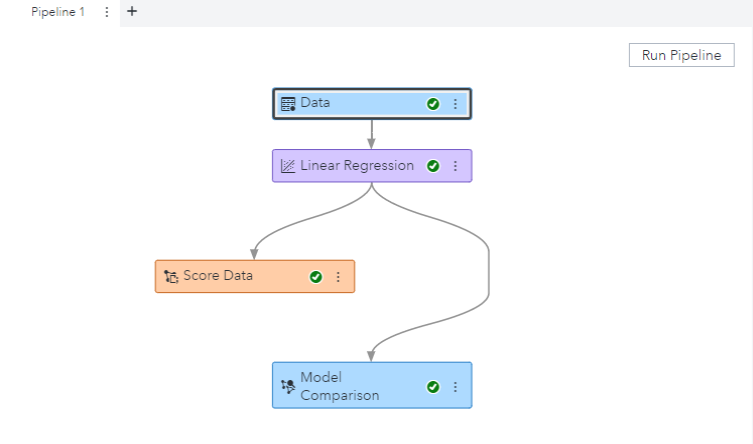Once the pipeline completes, and reviewing the initial results, it seems the model is behaving in a proper way; but when I test specific operands where the result is zero, I realize the model has misgivings: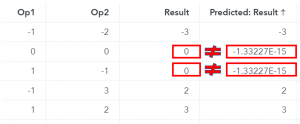Those are the operations with zero as result. I think that maybe, the algorithm hasn’t learned with the proper data, so I change the first seven registers of my initial dataset with the following operations with zeros: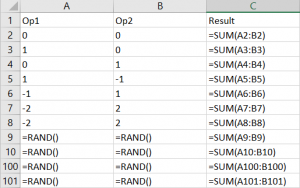Again, running the pipeline and letting the magic work, Voila!!!, the process has learned to handle the zeroes and properly sum two input numbers. When I check the results, I verify the results calculated (predicted) by the model are the same as the original ones that were indicated in the training and test dataset. So now, I am sure my new model is ready for use as my calculator compute engine.

Now that I have my compute engine (model) ready, it’s time to use it. We know it can perform the sum operation perfectly, so how do we perform the rest of the operations? We’ll take the sum model, move it into SAS Intelligent Decisioning, and add rules to handle the rest of the operations.

First, let’s explore the logic that will build the operations from our original model. This is where mathematics start playing in (Step 2). After exploring the operations we'll look at the Decision model where we'll define the logic to run the operations (Step 3).

Execute the model with the two input numbers, with no additional logic.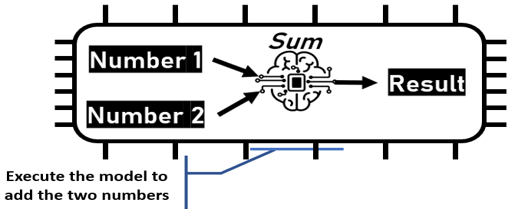### Subtraction

By changing the sign of the second input, the model does the rest.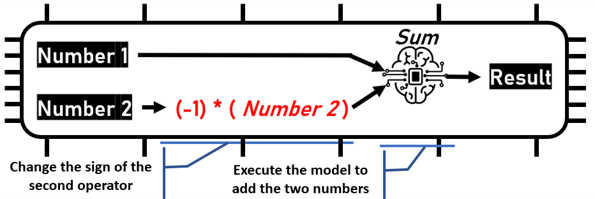That’s a simple enough solution for subtraction, but how do we handle multiplication and division? Let’s take a look.

### Multiplication and division

How can I perform a multiplication or a division operation if my compute engine only executes the addition operation? Here we can apply the magic of logarithmic properties stating:

• log (x*y) = log(x) + log(y)
• log (x/y) = log(x) – log(y)

Following this logic, if I want to multiply two numbers, I calculate the logarithm of each one and perform the addition operation in my compute engine. I follow this up by applying the exponential function to reverse the logarithm. The image below outlines the workflow.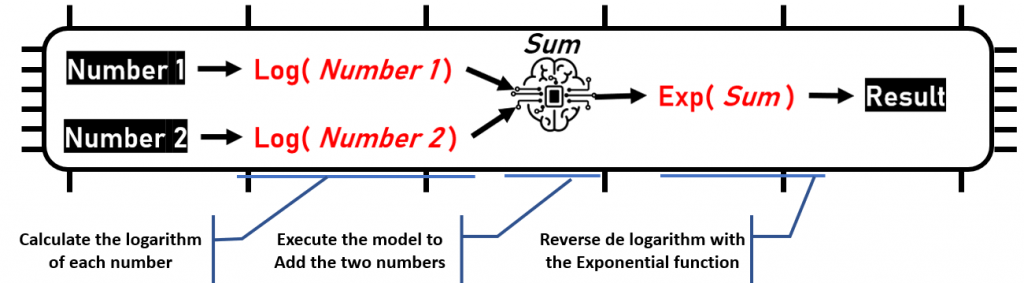For the division, it is the same process, but changing the sign of the second logarithm to the subtraction operation.

There are also certain cases requiring special treatment. For example, a division by zero generates an error, a logarithm of zero or a negative number cannot be calculated, and the multiplication of two numbers is zero if at least one of them is zero.

Let's now build the calculator in SAS Intelligent Decisioning, including the operations, the model, and the extra logic.

## Step 3 - Embed the needed logic into the decision to perform the calculator operations

The diagram below represents the Decision flow for our calculator example. Each node is numbered and followed by a definition.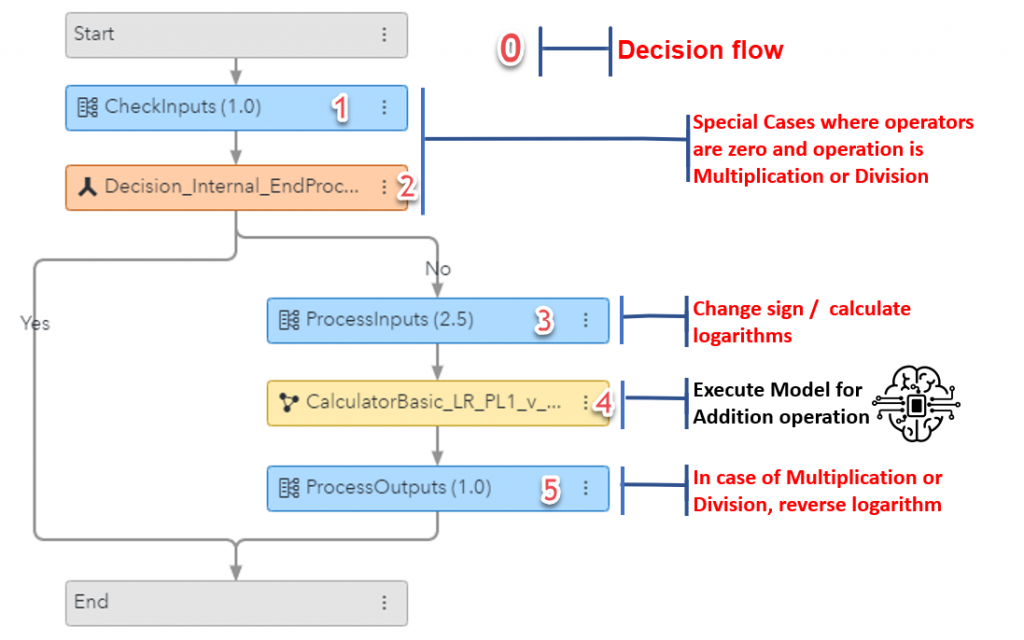0 - Overall decision flow - definition doc found here on GitHub
1 - Determine if zero is a factor for multiplication or division operations - definition doc found here on GitHub
2 - Decision handles value of previous step - set Variable = Decision_Internal_EndProcess = True
3 - Process calculations based on operation value - definition doc found here on GitHub
4 - Calculator linear regression model created earlier in the post - model definition file found here on GitHub
5 - Additional logic to finalize processing on multiplication and division operations - definition doc found here on GitHub

## Step 4 - Publish the final artifact created as an API service

After completing all the work on the decision, click on the Publish button and the Calculator is ready to be consumed via an API.

A colleague of mine created a simple web application which calls models using SAS Viya microservice APIs. I'll use this web app to display the results of my calculator. For brevity, I won't cover the details of the app. If you'd like to see how to create a web app using SAS APIs, I recommend the Build a web application using SAS Compute Server series on the SAS Communities.

The app allows me to choose my decision flow, add my operands and indicate an operation as seen below.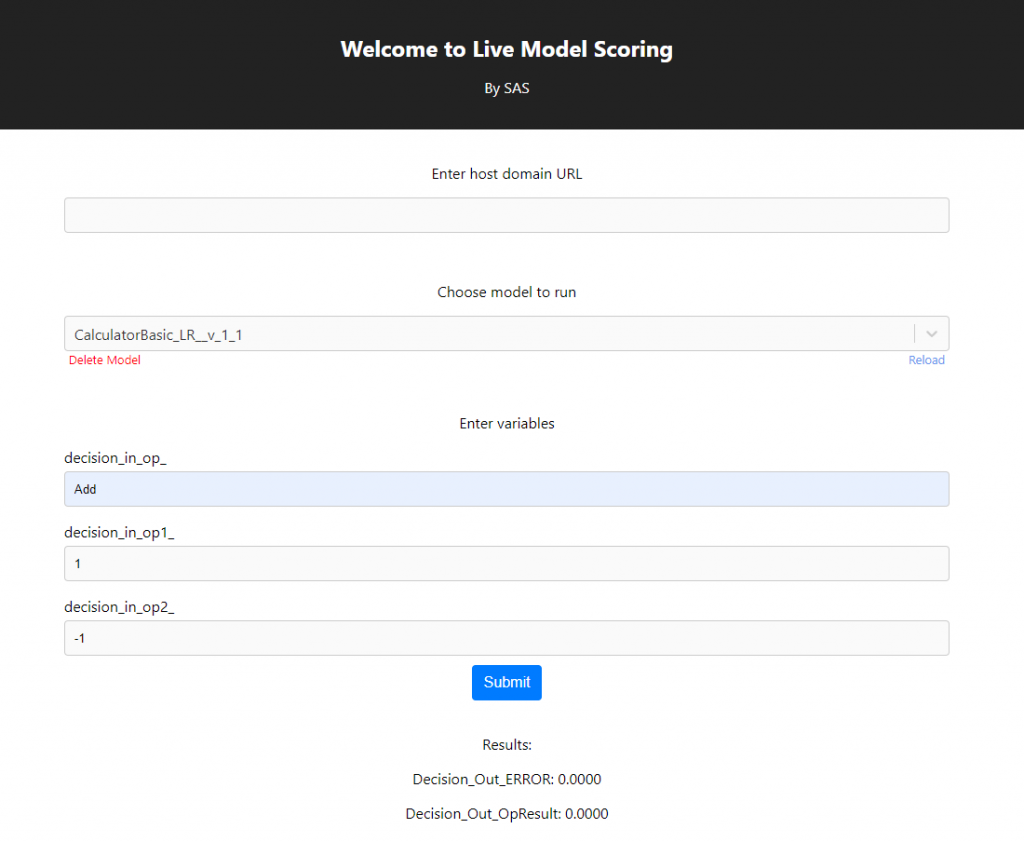I tested with several operand and operation combinations and they all checked out. It worked!

# Final Thoughts

I can consider my self-challenge solved. Through this example we accomplished the following:

• The Calculator API Service can perform the four operations based on a Machine Learning Model.
• I created a simple Machine Learning Model to perform the addition of two decimal numbers from a 100 registers data set.
• The model and the extra logic needed to perform the operations was developed under the low code / no code paradigm.
• I used the visual interface to generate the model and the extra logic, in conjunction with the expression builder, to apply the logarithm and exponential operations.
• The overall process has taken no more than a couple of hours.

Apart from the usefulness of this API, my principal takeaways of this self-challenge are:

• In this case, building my data set to obtain the exact behavior I wanted for my model was quite straight-forward.
• Building the model through the Graphical User Interface was easy and fast.
• Having the capacity to embed the models with extra logic under the low code / no code paradigm provides “supercharged intelligence” to the model
• The publishing feature of the whole artifact as an API service is great, providing instant value to the consumers.
• SAS Viya is a great platform as it provides all the pieces needed to satisfy your analytical business needs as well as your “curiosity needs”.

Share